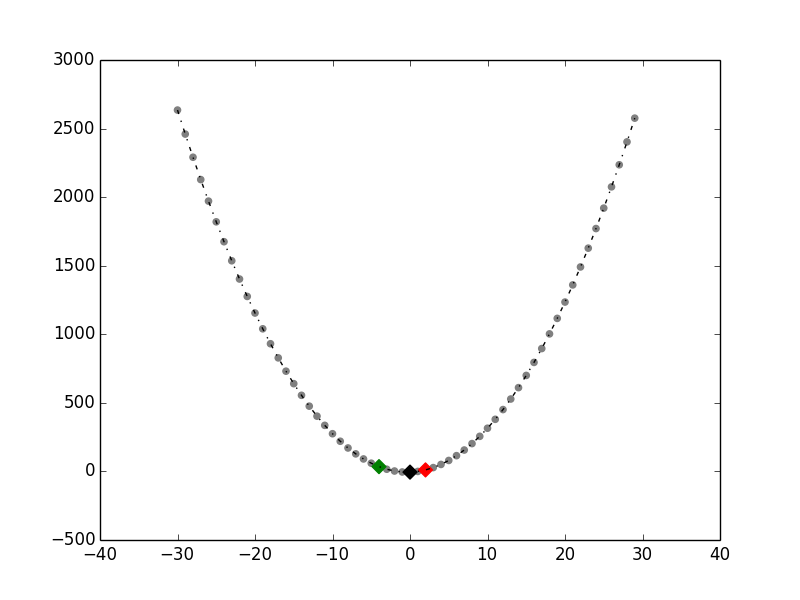# Calculate a parabola from three known points

I was looking for a quick fix for calculating values along a parabola given three known points. Scouting around I found a nice symbolic C function doing exactly what I wanted (see here). The function doing the work of finding your unknowns is:

By defining our three known xy points, we can use the above function to find the values of the unknowns a, b and c which would satisfy the equation of y = ax2 + bx + c:

We now have our full equation and can apply it to calc values along the parabola:

And then to plot it we can just do this:

…which gives us this:Written on November 16, 2015